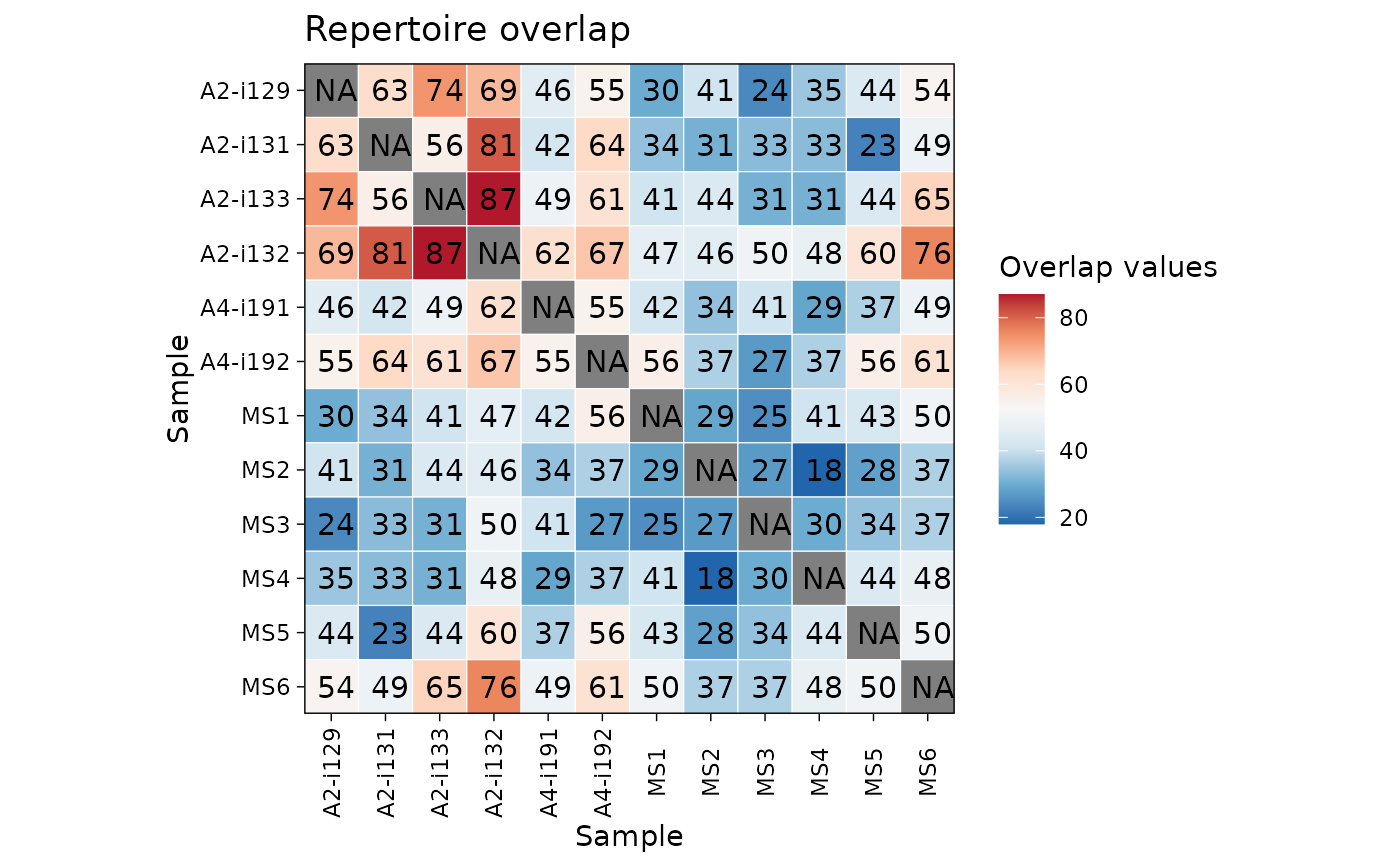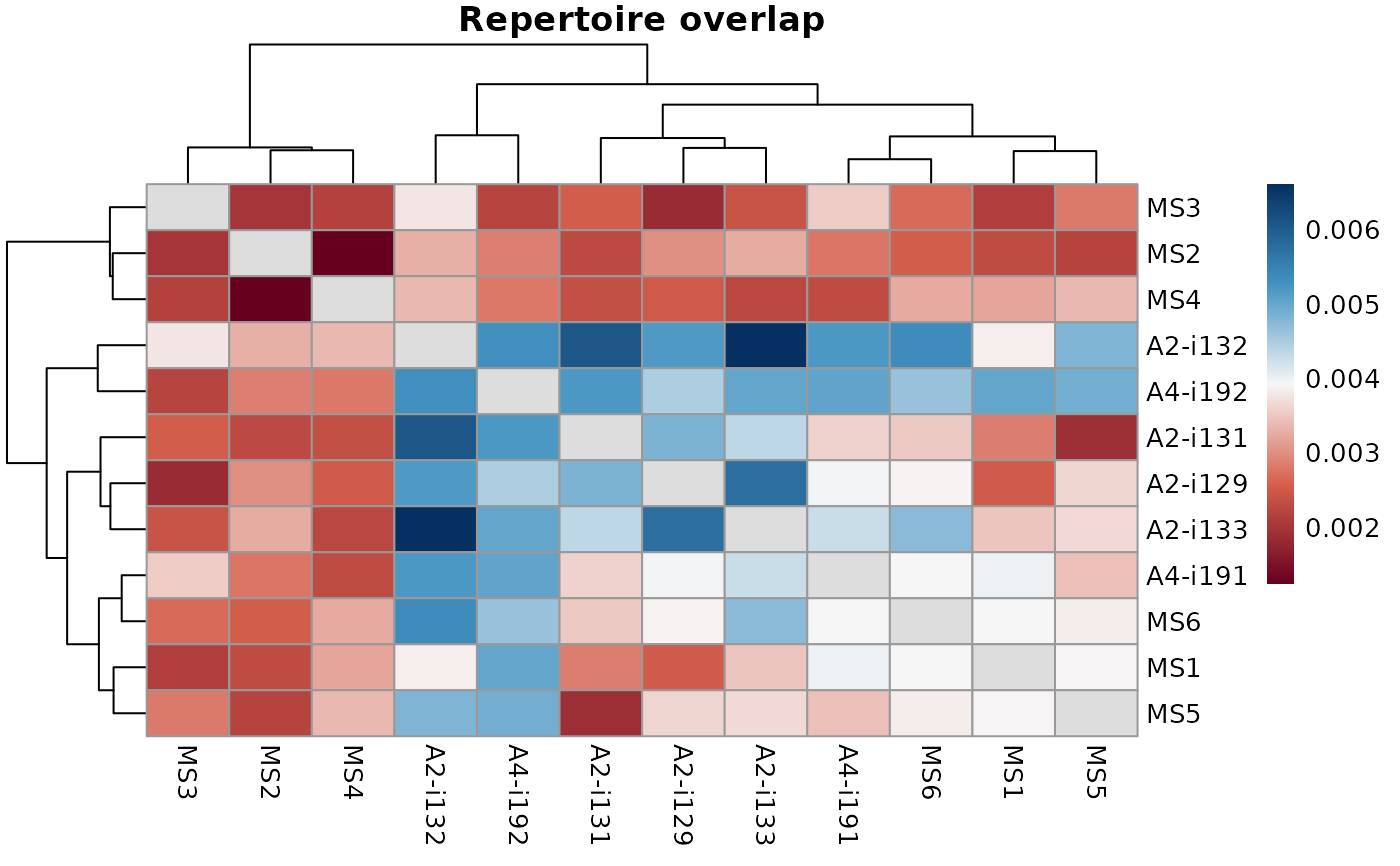The repOverlap function is designed to analyse the overlap between two or more repertoires. It contains a number of methods to compare immune receptor sequences that are shared between individuals.

repOverlap(
.data,
.method = c("public", "overlap", "jaccard", "tversky", "cosine", "morisita",
"inc+public", "inc+morisita"),
.col = "aa",
.a = 0.5,
.b = 0.5,
.verbose = TRUE,
.step = 1000,
.n.steps = 10,
.downsample = FALSE,
.bootstrap = NA,
.verbose.inc = NA,
.force.matrix = FALSE
)

## Arguments

.data

The data to be processed. Can be data.frame, data.table, or a list of these objects.

Every object must have columns in the immunarch compatible format. immunarch_data_format

Competent users may provide advanced data representations: DBI database connections, Apache Spark DataFrame from copy_to or a list of these objects. They are supported with the same limitations as basic objects.

Note: each connection must represent a separate repertoire.

.method

A string that specifies the method of analysis or a combination of methods. The repOverlap function supports following basic methods: "public", "overlap", "jaccard", "tversky", "cosine", "morisita". If vector of multiple methods is given for this parameter, the first method will be used.

.col

A string that specifies the column(s) to be processed. Pass one of the following strings, separated by the plus sign: "nt" for nucleotide sequences, "aa" for amino acid sequences, "v" for V gene segments, "j" for J gene segments. E.g., pass "aa+v" to compute overlaps on CDR3 amino acid sequences paired with V gene segments, i.e., in this case a unique clonotype is a pair of CDR3 amino acid and V gene segment. Clonal counts of equal clonotypes will be summed up.

.a, .b

Alpha and beta parameters for Tversky Index. Default values give the Jaccard index measure.

.verbose

if TRUE then output the progress.

.step

Either an integer or a numeric vector.

In the first case, the integer defines the step of incremental overlap.

In the second case, the vector encodes all repertoire sampling depths.

.n.steps

Skipped if ".step" is a numeric vector.

.downsample

If TRUE then performs downsampling to N clonotypes at each step instead of choosing the top N clonotypes in incremental overlaps. Change nothing of you are using conventional methods.

.bootstrap

Set NA to turn off any bootstrapping, set a number to perform bootstrapping with this number of tries.

.verbose.inc

Logical. If TRUE then shows output from the computation process.

.force.matrix

Logical. If TRUE then always forces the matrix output even in case of two input repertoires.

## Value

In most cases the return value is a matrix with overlap values for each pair of repertoires.

If only two repertoires were provided, return value is single numeric value.

If one of the incremental method is chosen, return list of overlap matrix.

## Details

"public" and "shared" are synonyms that exist for the convenience of researchers.

The "overlap" coefficient is a similarity measure that measures the overlap between two finite sets.

The "jaccard" index is conceptually a percentage of how many objects two sets have in common out of how many objects they have total.

The "tversky" index is an asymmetric similarity measure on sets that compares a variant to a prototype.

The "cosine" index is a measure of similarity between two non-zero vectors of an inner product space that measures the cosine of the angle between them.

The "morisita" index measures how many times it is more likely to randomly select two sampled points from the same quadrat (the dataset is covered by a regular grid of changing size) then it would be in the case of a random distribution generated from a Poisson process. Duplicate objects are merged with their counts are summed up.

data(immdata)
immdata$data <- top(immdata$data, 4000)
ov <- repOverlap(immdata$data, .verbose = FALSE) vis(ov)ov <- repOverlap(immdata$data, "jaccard", .verbose = FALSE)